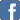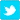# Format Number with Decimals Without Rounding

Posted on the 31 October 2012 by Akahgy

Description:

Trying to get a precise number of decimal in a number results most of the times in a rounding of the number. Both round() and number_format() functions of PhP round the output result.

Solution:

The solution to this issue is to multiply the number by the power of 10 that is the number of decimals. 10 for 1 digit, 100 for 2 digits and so on.

\$originalNumber = 150.8699; //we want to obtain 150.86
\$multipliedNumber = originalNumber * 100; //output is 15086.99

Then, we apply the floor() function, to get rid of the additional trailing decimals:

\$integerMultipliedNumber = floor(\$multipliedNumber); //output is 15086

Finally, divide the number back to the decimal power of 10 (100 in our case) and parse it as a float:

\$twoDecimalResult = (float) (\$integerMultipliedNumber / 100); //output is 150.86